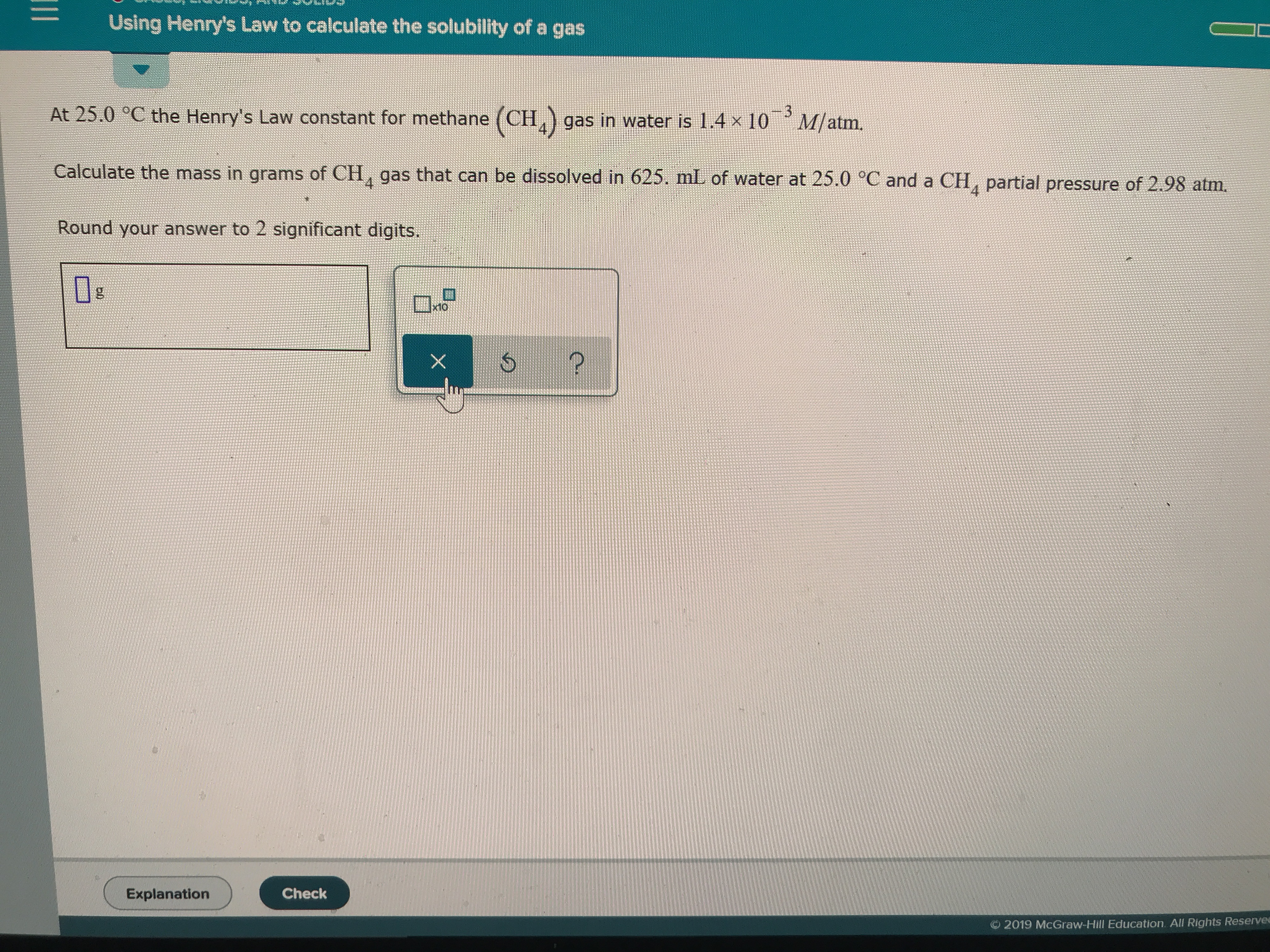# Using Henry's Law to calculate the solubility of a gas At 25.0 °C the Henry's Law constant for methane (CH,) gas in water is 1.4 x 10 M/atm. 3 Calculate the mass in grams of CH, gas that can be dissolved in 625. mL of water at 25.0 °C and a CH, partial pressure of 2.98 atm. 4 Round your answer to 2 significant digits x10 X Explanation Check 2019 McGraw-Hill Education. All Rights Reservee

Question

Calculate the mass in grams of CH4 gas that can be dissolved in 625.  ML of water at 25 Celsius and a CH 4 partial pressure of 2.98 ATM.help_outlineImage TranscriptioncloseUsing Henry's Law to calculate the solubility of a gas At 25.0 °C the Henry's Law constant for methane (CH,) gas in water is 1.4 x 10 M/atm. 3 Calculate the mass in grams of CH, gas that can be dissolved in 625. mL of water at 25.0 °C and a CH, partial pressure of 2.98 atm. 4 Round your answer to 2 significant digits x10 X Explanation Check 2019 McGraw-Hill Education. All Rights Reservee fullscreen

### Want to see this answer and more?

Experts are waiting 24/7 to provide step-by-step solutions in as fast as 30 minutes!*

*Response times vary by subject and question complexity. Median response time is 34 minutes and may be longer for new subjects.
Tagged in
ScienceChemistry

### Physical Chemistry